Polar Coordinates

(requires JavaScript)

1. Sketch the region in the plane consisting of points whose polar coordinates satisfy $-1\le r\le 1$ and $\frac{\pi }{4}\le \theta \le \frac{3\pi }{4}$.

2. Find a polar equation for the curve represented by the Cartesian equation

${x}^{2}+{y}^{2}=2cx$

$r=2c\mathrm{cos}\left(\theta \right)$
3. Sketch the curve given by $r=\mathrm{sin}\left(\theta \right)$.
A circle through the origin and $\left(1,\frac{\pi }{2}\right)$.
4. Sketch the curve given by $r=2\mathrm{cos}\left(4\theta \right)$.
5. Match the polar equations with the graphs without using a computer. Explain.

1. $r=\mathrm{sin}\left(\frac{\theta }{2}\right)$
2. $r=\mathrm{sin}\left(\frac{\theta }{4}\right)$
3. $r=\frac{1}{\mathrm{cos}\left(3\theta \right)}$
4. $r=\theta \mathrm{sin}\left(\theta \right)$
5. $r=1+4\mathrm{cos}\left(5\theta \right)$
6. $r=\frac{1}{\sqrt{\theta }}$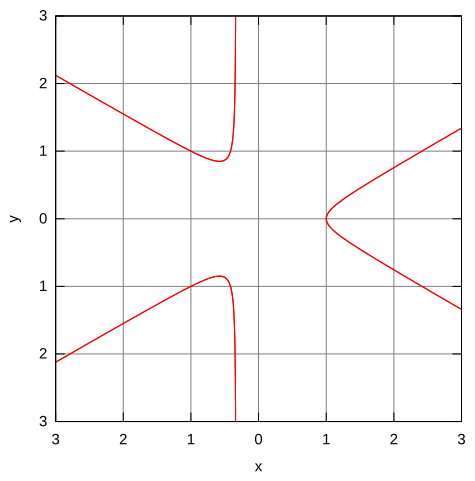(a)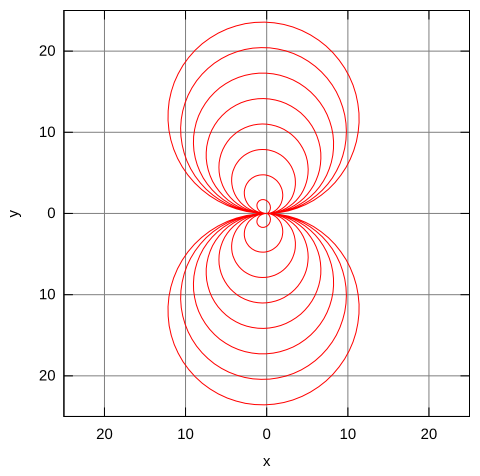(b)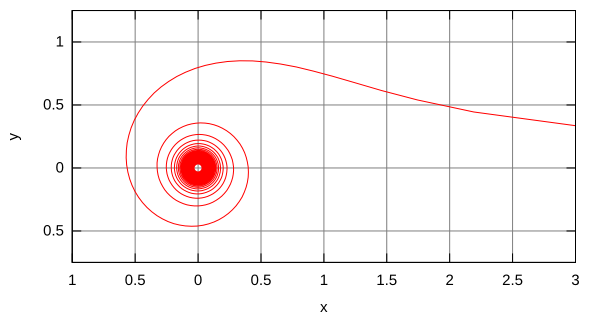(c)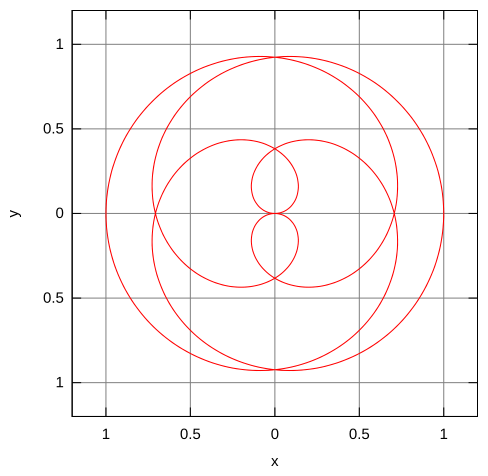(d)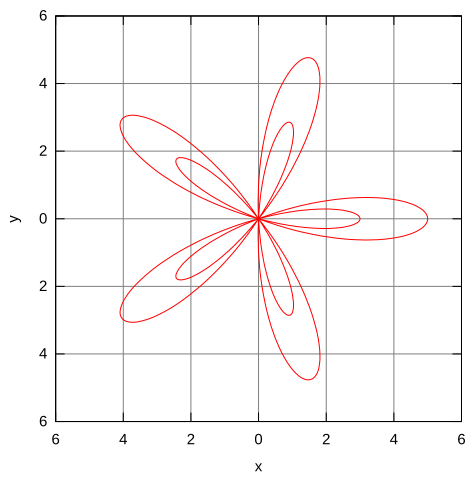(e)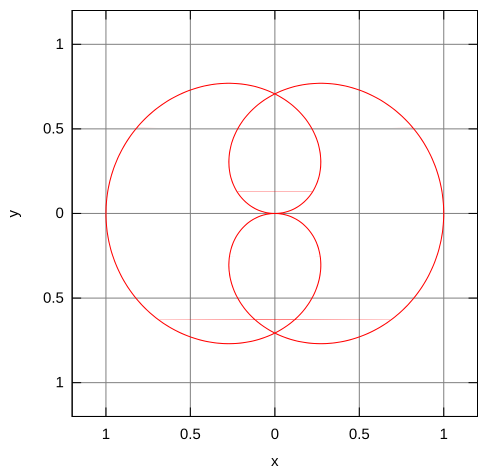(f)